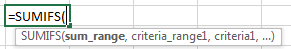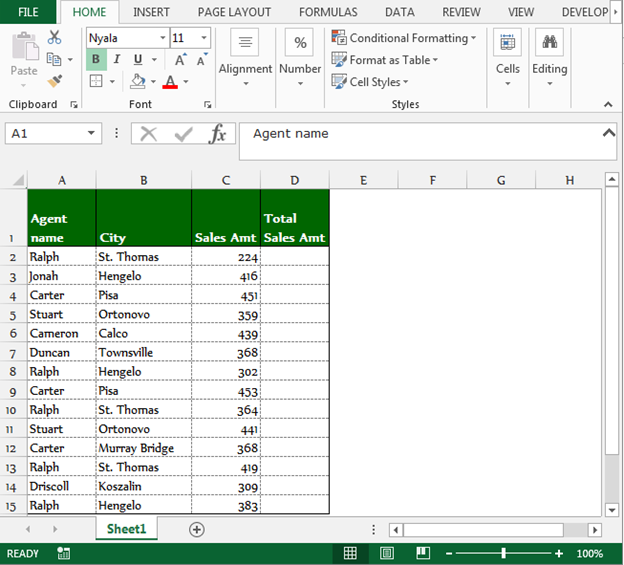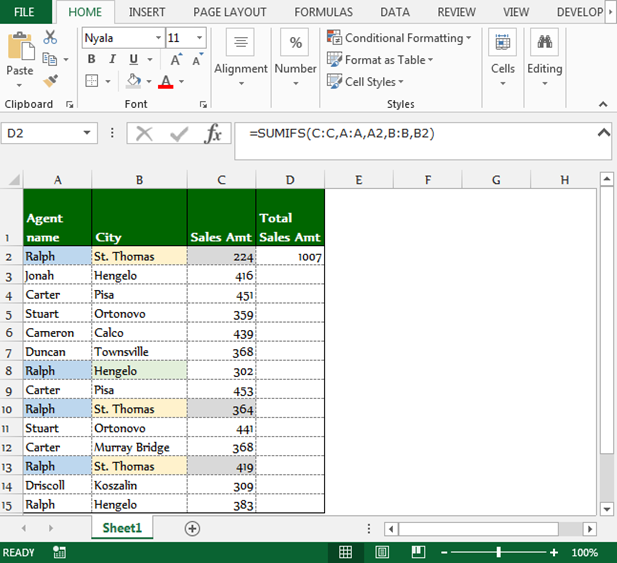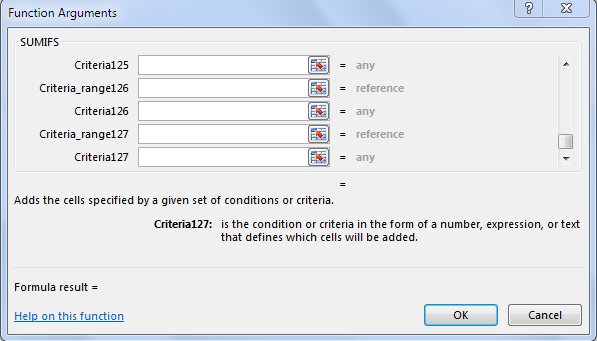# Summing using multiple criteria in Microsoft Excel

In this article you will learn how to sum using multiple criteria in Microsoft Excel.

SUMIFS:This function is used for adding the cells specified by a given set of condition or criteria.Let’s take an example to understand how we can use the SUMIFS function.

We have sales data in range A1:C15. Column A contains agent's name, column B contains City name, and column C contains sales amount.In the picture shown above, we have data in excel like this. We want to return the total value of every agent, and agent should be from the same city.

To return the total value agent wise, follow below given steps:-

• Select the cell D2.
• Write the formula.
• =SUMIFS(C:C,A:A,A2,B:B,B2)press enter on your keyboard.
• The function will return the total value for the agent Ralph and his city St. Thomas.To return the total amount for all the agents, copy the same formula by pressing the key Ctrl+C and paste in the range D3:D15 by pressing the key Ctrl+V on your keyboard.

Note:- Through SUMIFS function, we can check 127 criteria in a cell. Use SUMIFS function to sum the values for multiple criteria.